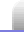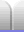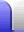# Messing With Infinity

### Overview

Infinity is not really a number, but more of a concept. Because it's not really a number, we have to be careful about how we use it.

When we start to do stuff with infinity, strange things can happen. For example, positive infinity can appear to be the same as negative infinity, as seen in the tangent function at 90°. We can also get very useful results.

### 0.9 ≡ 1

0.9 is a recurring number. The string of 9s carries on forever. Because it never stops, 0.9 is exactly the same as 1. This is also mentioned in the rational numbers section on the Number Sets page.

1/3 = 0.333...

2/3 = 0.666...

3/3 = 0.999...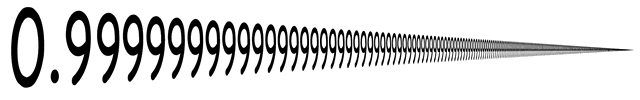∴ 0.999... ≡ 1

### The (at least) four values of 0 / 0

The fraction made by zero divided by zero is a curious one. Taking a simplified view of fractions, we appear to have several basics that clash with each other.

• Zero divided by any number is zero.

0 / 0 = 0

• Any number divided by itself is 1 (a unity fraction).

0 / 0 = 1

• Any number divided by zero is infinity.
• (As a fraction's denominator approaches zero the value of the fraction aproaches infinity.)

0 / 0 = ∞

• Zero is neither positive or negative.
• (This means that as a fraction's negative denominator approaches zero the value of the fraction aproaches negative infinity.)

0 / 0 = -∞

This implies that 00 is 0, 1, ∞, and -∞ all at the same time. It is not possible for a simple numeric fraction to have four values at once.

0 / 0 = undefined

00 can also be engineered to have other values. For more information about what 00 means and what it's useful for, see this video.

### 0 = ∞ = 1

Let's say we have a number x, such that x is the sum of all natural numbers to infinity.

x = 1 + 2 + 3 + 4 + 5 + ...

Let's add a zero to both sides.

0 + x = x = 0 + 1 + 2 + 3 + 4 + 5 + ...

And now we'll substract the second equation from the first.

x - x = 1 + 1 + 1 + 1 + 1 + ...
⇒ 0 = 1 + 1 + 1 + 1 + 1 + ...
0 = ∞

This itself is nonsensical, because it's saying zero equals infinity, but we'll continue. Let's add a zero to both sides again.

0 = 0 + 1 + 1 + 1 + 1 + 1 + ...

And subtract the last equation from the second to last.

0 - 0 = 1 + 0 + 0 + 0 + 0 + 0 + ...
0 = 1

Hence, zero equals one. Not because it actually does, but because we messed with infinity (by substracting infinity from infinity).

### 1∞

This one is a bit more subtle. At first glance, 1 multiplied by itself any number of times will never be anything but 1. Actually, it can be 1, but it's not necessarily so.

Let's assume 1 does equal 1, and see what happens when we take the logarithm of both sides. (It doesn't matter which logarithm base we use.)

1 = 1
log( 1 ) = log(1)
∞·log(1) = 0
∞·0 = 0
∞ = 00

As we saw above, infinity is only one value that 00 can take, so 1 is a value 1 can take but it's not the only value. Why is this?

Remember, infinity is not a number, which means 1 is not a number either, but is actually referring to some sort of limiting process, which the exponent and possibly the base are subject to.If we are specifically talking about the limit of 1x as x → ∞ then that limit is 1.If the base is also subject to the limit then we have to take the limits of the two functions at the same time. Depending on whether the base or the exponent converges more quickly, we might end up with 0 or infinity, or anything in between. For example, e.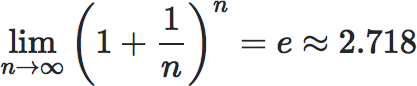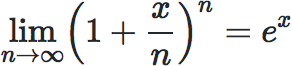FWIW that second one is the actual calculation that a computer or calculater does when you ask it to work out what ex is.

In summary, 1 actually represents a limit and can have any value. Be careful when messing with infinity, and with limits.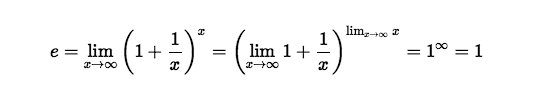Home Astronomy Chemistry Electronics & Computers Mathematics Physics Field Trips Turn on javascript for email link